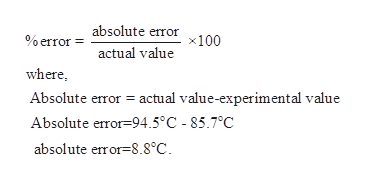# How do we calculate the percent error for melting point for an experiment where we got a melting point of benzil of 83-88.4C?And we know that the known and tested melting point of benzil is 94-95C.

Question
134 views

How do we calculate the percent error for melting point for an experiment where we got a melting point of benzil of 83-88.4C?

And we know that the known and tested melting point of benzil is 94-95C.

check_circle

Step 1

This problem can be solve using the formula shown below.help_outlineImage Transcriptionclose% errorabsolute error 100 actual value where Absolute error actual value-experimental value Absolute error-94.5°C - 85.7°C absolute error=8.8°C. fullscreen
Step 2

Knowing the absolute error, % error can be calculated using the formula described...

### Want to see the full answer?

See Solution

#### Want to see this answer and more?

Solutions are written by subject experts who are available 24/7. Questions are typically answered within 1 hour.*

See Solution
*Response times may vary by subject and question.
Tagged in

### Physical Chemistry